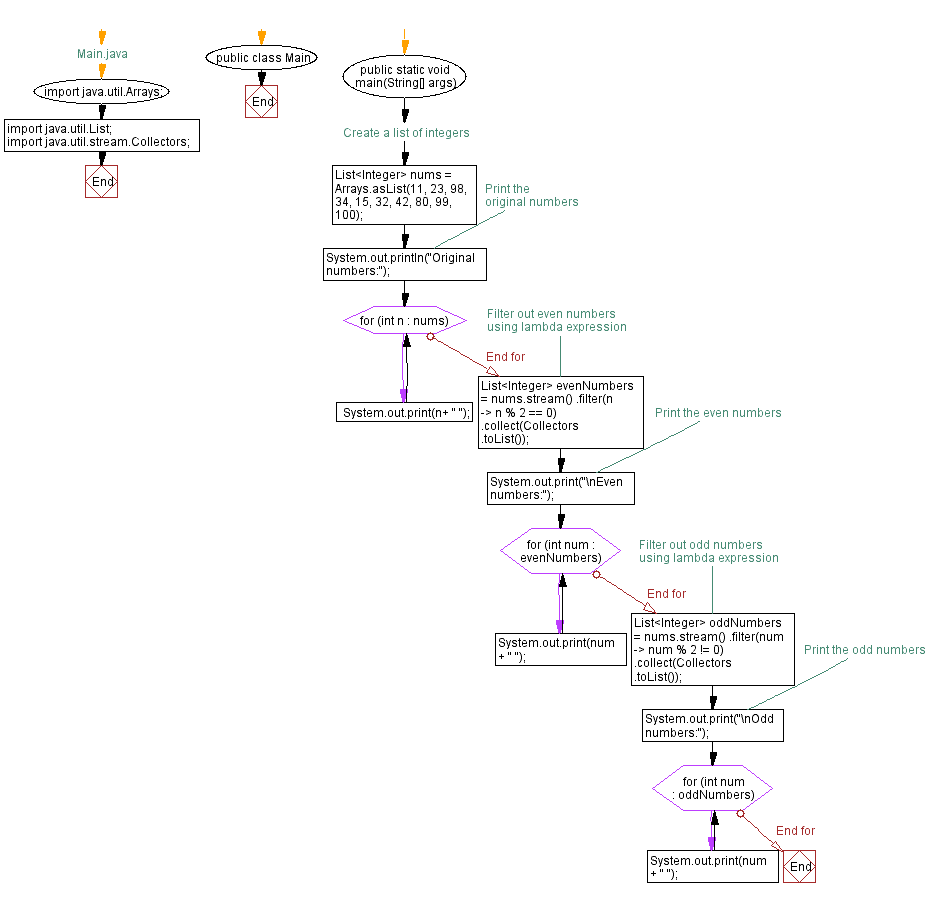# Filter even and odd numbers from list using Lambda expression in Java

## Java Lambda Program: Exercise-4 with Solution

Write a Java program to implement a lambda expression to filter out even and odd numbers from a list of integers.

Sample Solution:

Java Code:

``````// Main.java
import java.util.Arrays;
import java.util.List;
import java.util.stream.Collectors;

public class Main {
public static void main(String[] args) {
// Create a list of integers
List nums = Arrays.asList(11, 23, 98, 34, 15, 32, 42, 80, 99, 100);

// Print the original numbers
System.out.println("Original numbers:");
for (int n : nums) {
System.out.print(n+ " ");
}

// Filter out even numbers using lambda expression
List evenNumbers = nums.stream()
.filter(n -> n % 2 == 0)
.collect(Collectors.toList());

// Print the even numbers
System.out.print("\nEven numbers:");
for (int num : evenNumbers) {
System.out.print(num + " ");
}

// Filter out odd numbers using lambda expression
List oddNumbers = nums.stream()
.filter(num -> num % 2 != 0)
.collect(Collectors.toList());

// Print the odd numbers
System.out.print("\nOdd numbers:");
for (int num : oddNumbers) {
System.out.print(num + " ");
}
}
}
``````

Sample Output:

```Original numbers:
11 23 98 34 15 32 42 80 99 100
Even numbers:98 34 32 42 80 100
Odd numbers:11 23 15 99
```

Explanation:

At first create a list of integers called nums using the Arrays.asList() method and print the original list.

To filter out even numbers, we use the stream() method on the list nums to convert it into a stream. Now apply a lambda expression with the filter() method. The lambda expression (n -> n % 2 == 0) checks if a number is divisible by 2 (i.e., even). We collect the filtered even numbers into a new list using the collect() method with Collectors.toList().

To filter out odd numbers, we use a similar approach. We again use the stream() method on the nums list, apply a lambda expression (num -> num % 2 != 0) to check if a number is not divisible by 2 (i.e., odd), and collect the filtered odd numbers into a new list using collect() and Collectors.toList().

Flowchart:Live Demo:

Java Code Editor:

Improve this sample solution and post your code through Disqus

Java Lambda Exercises Previous: Convert list of strings to uppercase and lowercase using Lambda expression in Java.
Java Lambda Exercises Next: Sort strings in alphabetical order using Lambda expression in Java.

What is the difficulty level of this exercise?

Test your Programming skills with w3resource's quiz.

﻿

## Java: Tips of the Day

Float vs double

Programmers often cannot select the precision they need for floating-point numbers. Float requires only 4 bytes, but it has only 7 significant digits, while Double is twice as accurate (15 digits), but twice as wasteful.

In fact, most processors are able to work with Float and Double equally effectively, so use the recommendation of Bjorn Stroustrup:

"Choosing the right accuracy in real-world problems requires a good understanding of the nature of machine computing. If you do not have it, either consult with someone, or study the problem yourself, or use double and hope for the best."

Ref: https://bit.ly/3y3CjL9

We are closing our Disqus commenting system for some maintenanace issues. You may write to us at reach[at]yahoo[dot]com or visit us at Facebook